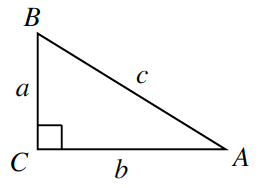### Home > INT3 > Chapter 12 > Lesson 12.1.4 > Problem12-58

12-58.

Using the triangle at right, determine:

1. $\operatorname { sin } ( A )$

Remember that $\sin(x)$ is opposite over hypotenuse.

$\sin\left(A\right)=\frac{a}{c}$

1. $\operatorname { csc } ( A )$

Which trigonometric ratio is $\csc(x)$ related to? How is it related?

1. $\cos(B)$

1. $\sec(B)$

How is $\sec(x)$ related to $\cos(x)$?